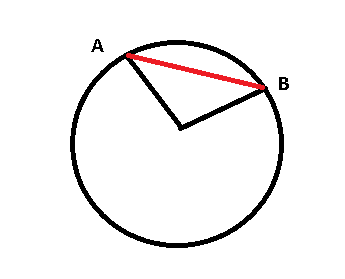# Mathematical proof (Drawing a help line)

• Dousin12
In summary, the conversation discusses a proof being done in school using mathematical language and symbols. The person needs help writing a line connecting two points in a neat mathematical way and others suggest using notations such as "crd theta" or "chord AB". There is also a discussion about different notations for angles and the difference between them.

## Homework Statement

I'm doing quite a strict proof in school. Where we should proof something and use mathematical language and symbols.

## Homework Equations## The Attempt at a Solution

To proof what I have to proof I need to draw some help lines. As for instance the "red" one I did from A to B. However I'm very unsure how to write this in mathematical symbols that I draw a helpline to form a triangle ABC (If you imagine that the intersection between A and B is called C.

Any tips on how to write this in a neet mathematical way?

Last edited by a moderator:
The line connecting A and B is called chord. According to this, you can write it as ##\textrm{crd }\theta## where ##\theta## is the sector angle subtending the chord. Nevertheless, I think you can also denote it as ##\overline{AB}##.

The AB written with a line over is the angle that that the "ARC" ab forms with the midpoint. So I think the crd theta is better option here.

Thanks a lot for the help man :)

(If other people think otherwise they can also reply if you want :P)

I might need to write it without using "crd theta" though. Because I don't think I will use the theta angle. I more want to write "I draw the help line.. to form a triangle"

Dousin12 said:
The AB written with a line over is the angle that that the "ARC" ab forms with the midpoint.
If I am allowed to be more pedantic, I should deny that because as you can see in this link, an arc between two points looks like the way it's shown there.
Dousin12 said:
I more want to write "I draw the help line.. to form a triangle"
IMO, saying "chord AB" will not create an ambiguous meaning in your writing.

" Consider an imaginary line drawn between points A and B . If this line is bisected at point C then ...

I have never seen chord or crd, interesting though. The notations I know in geometry are ##\overline{AB}## for the straight through ##A## and ##B## or ##\overline{ABD}## if there is another point ##D## on it, ##\stackrel{\mbox{$\frown$}}{AB}## for the arc between them and ##\angle{ACB}, \; \sphericalangle{ACB} ## or ##\measuredangle{ACB}## for the angle at ##C## between the straights ##\overline{CA}## and ##\overline{CB}##. Parallels are ##\overline{AD}\; \| \; \overline{CB}## and ##\perp## denotes perpendicularity.

fresh_42 said:
∠ACB,∢ACB∠ACB,∢ACB\angle{ACB}, \; \sphericalangle{ACB} or ∡ACB

What is the difference between these 3 notations for angles?

Dousin12 said:
What is the difference between these 3 notations for angles?
None. Simply a matter of taste. I like the second one, the first might be for the lazy. However, I've seen the first one often in technical drawings.